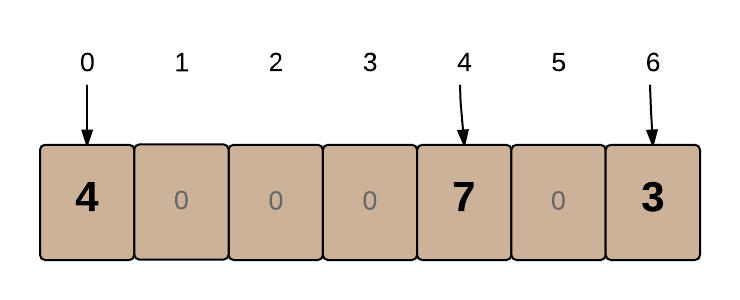# HashTables

Maps and Sets provide a clean interface for accessing or looking up the elements within them. There are a number of different ways in which they can be implemented, but the most common one uses a technique known as hashing.

Q: What is Hashing and what makes it so special?
A: A program usually needs to check through multiple elements in a collection to find a single element, but hashing lets it find items immediately, regardless of the size of the collection!
Q: How does hashing do that?
A: Let's see by implementing a basic HashTable, the hashing structure behind HashSets and HashMaps.

### Implementing a HashTable

Let's say you have a set of integer keys which map to values and you want to create a structure that can find and modify the values in constant time.

If your set of keys was just a small range of integers, a HashMap would not be necessary since you could use a simple array to store the values, with the index values of the array as 'keys':In the above array, 0 'maps' to 4 and 4 'maps' to 7. You can create a new 'mapping' for any key `k` (where `k` is less than the array's length) by simply updating kth cell in the array with the value you want.1

However, if the range of keys is large, an array would need to use up a lot of memory in order to store everything. For example, it would be very inefficient to create an Array of length 200M (200 Million) just to store the following 7 items:

`2, 341, 73, 8265, 234004, 7.4M, 200M`

Mini-challenge: Can you think of a simple way to convert the above data so it can be stored in a small array, say of size 10?

Solution
We can use the mod operator `%` on a number to get a remainder `r` and store the element in index `r` of the array. For example, if we did `mod 10` on each number, we would get the following remainders: